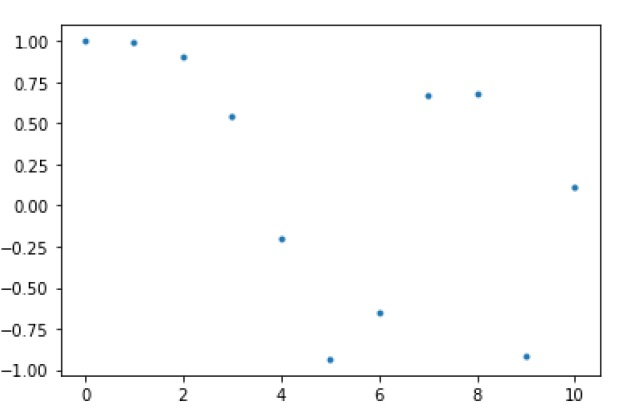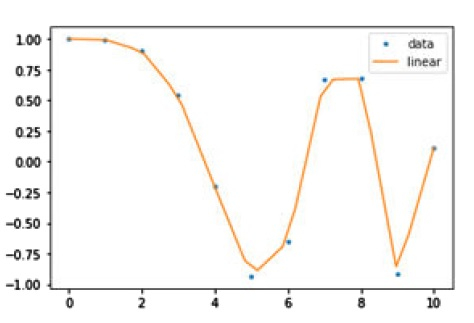# What is interpolation and how can we implement it in the SciPy Python library?

Interpolation is a method of generating a value between two given points on a line or a curve. In machine learning, interpolation is used to substitute the missing values in a dataset. This method of filling the missing values is called imputation. Another important use of interpolation is to smooth the discrete points in a dataset.

SciPy provides us a module named scipy.interpolate having many functions with the help of which we can implement interpolation.

## Example

In the below example we will implement Interpolation by using the scipy.interpolate() package −

First let’s generate some data to implement interpolation on that −

import numpy as np
from scipy.interpolate import interp1d
import matplotlib.pyplot as plt
A = np.linspace(0, 10, num=11, endpoint=True)
B = np.cos(-A**2/9.0)
print (A, B)

## Output

The above script will generate the following points between 0 and 4 −

[ 0. 1. 2. 3. 4. 5. 6. 7. 8. 9. 10.] [ 1. 0.99383351 0.90284967 0.54030231 -0.20550672 -0.93454613
-0.65364362 0.6683999 0.67640492 -0.91113026 0.11527995]

Now, let’s plot these points as follows

plt.plot(A, B, '.')
plt.show()Now we need to create a interpolate function based on fixed data points. Let’s create it −

function_interpolate = interp1d(A, B, kind = 'linear')


To see the clear difference of interpolation, we will create a new input of more length by using the same function as used for old input −

Anew = np.linspace(0, 10, num=30, endpoint=True)
plt.plot(A, B, '.', Anew, function_interpolate(Anew), '-')
plt.legend(['data', 'linear'], loc = 'best')
plt.show()